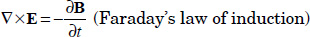# 2.1. Theoretical background

The following four Maxwell’s equations can describe all electromagnetic phenomena such as radiowaves and light:

[2.1][2.2][2.3][2.4]where H, B = μH, E, D = εE, J = σE and ρ indicate magnetic field (A/m), magnetic flux density (T), electric field (V/m), electric flux density (C/m2), current density (A/m2) and charge density (C/m3), respectively. μ, ε and σ indicate magnetic permeability (H/m), permeability (F/m) and electrical conductivity (1/Ω·m), respectively. Maxwell’s equations indicate that electromagnetic waves propagate in a field based on relationships between the electric field, magnetic field, time and space.

It is known that electromagnetic energy is also associated with the propagation of electromagnetic waves. The energy of electromagnetic waves can be described as E×H (W/m2). The vector E×H is called the Poynting vector. In plane wave propagation, the vector E×H results in a vector along the direction of propagation, which is same as the direction of the energy flow. It means that all electromagnetic waves themselves ...

Get Wireless Power Transfer via Radiowaves now with the O’Reilly learning platform.

O’Reilly members experience live online training, plus books, videos, and digital content from nearly 200 publishers.